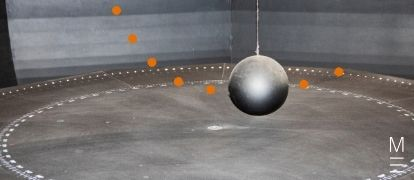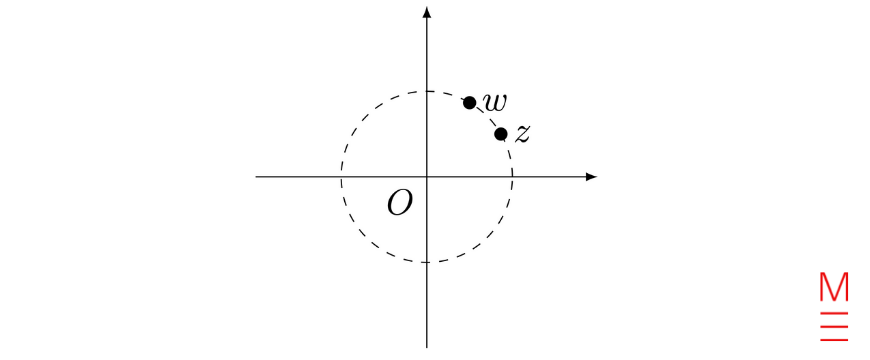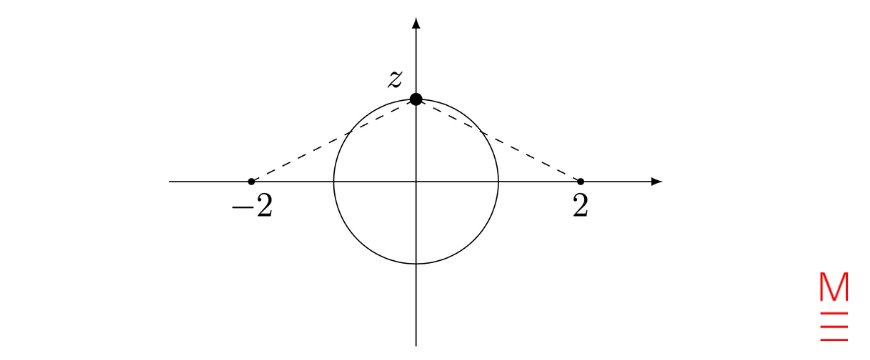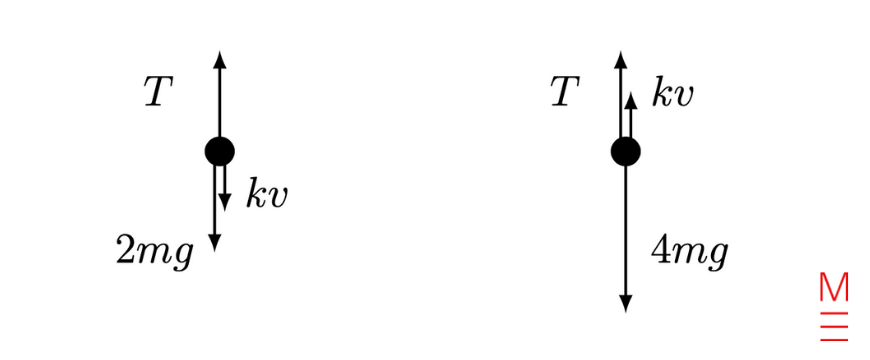# 2020 HSC Maths Ext 2 Exam Paper Solutions

In this post, we give you the solutions to the 2020 Maths Extension 2 paper.The Matrix 2020 HSC Maths Ext 2 Exam Paper Solutions are here!

## 2020 HSC Maths Ext 2 Exam Paper Solutions

Have you seen the 2020 HSC Mathematics Extension 2 exam Paper yet?

In this post, we will work our way through the 2020 HSC Maths Extension 2 exam paper and give you the solutions, written by our Head of Mathematics Oak Ukrit and his team.

Read on to see how to answer all of the 2020 questions.

## Section 1. Multiple Choice

 Question Number Answer Solution 1. B \begin{equation*} |-\boldsymbol{i} + 18\boldsymbol{j} -6 \boldsymbol{k}| = \sqrt{(-1)^2 + 18^2 + (-6)^2} = \sqrt{361} = 19  \\ \end{equation*} 2. B Since the quadratic $$P(z) = z^2 + pz + q$$ has real coefficients and $$z = 3+i$$ is a non-real root, the conjugate root theorem implies that $$\overline{3+i} = 3-i$$ is also a root of $$P(z)$$. Hence \begin{align*} -p &= \text{sum of roots } = (3+i) + (3-i) = 6 \\ q &= \text{product of roots } = (3+i)(3-i) = 10 \\ \end{align*} Hence $$p = -6, q = 10$$. 3. C We have $$x = 1 – 2 \lambda$$ and $$y = 3 + 4\lambda$$. Hence \begin{align*} \lambda = \frac{x-1}{-2} = \frac{y-3}{4} &\Rightarrow 4(x-1) = -2(y-3) \\ &\Rightarrow 4x + 2y – 10 = 0 \\ &\Rightarrow y + 2x = 5 \\ \end{align*} 4. A Let $$w = \displaystyle\frac{z^2}{|z|}$$. Then \begin{equation*} |w| = \left| \frac{z^2}{|z|} \right| = \frac{|z|^2}{|z|} = |z|\\ \end{equation*} so $$w$$ has the same modulus as $$z$$. Moreover, \begin{equation*} \arg(w) = \arg( \tfrac{z^2}{|z|} ) = \arg(1/|z|) + \arg(z^2) = 2 \arg(z) \\ \end{equation*} where we have used the fact that $$1/|z| > 0$$ (which implies $$\arg(1/|z|) = 0$$). Thus $$w$$ has twice the argument of $$z$$.5. D We use the formulas $$a_{\text{max}} = n^2 A$$ and $$v_{\text{max}} = nA$$. Then $$6 = n^2 A$$ and $$4 = nA$$, from which we deduce \begin{equation*} n = \frac{n^2 A}{nA} = \frac{6}{4} = \frac{3}{2} \\ \end{equation*} Therefore $$T = \frac{2\pi}{n} = \frac{4\pi}{3}$$ seconds. 6. A A routine integral. \begin{equation*} \int \frac{1}{x^2 + 4x + 10} \,dx = \int \frac{1}{(x+2)^2 + 6} \,dx = \frac{1}{\sqrt{6}} \tan^{-1} \left( \frac{x+2}{\sqrt{6}} \right) + C \\ \end{equation*} 7. D To disprove the statement, we need to find a prime number $$n$$ such that $$2^n – 1$$ is not prime. Thus the statement  “$$2^{11} – 1$$ is divisible by $$23$$” is a counterexample. 8. B The statement can be rewritten as: “If $$n$$ is even and a multiple of $$3$$, then $$n$$ is a multiple of $$6$$”. This has the structure $$P \Rightarrow Q$$. Therefore, to negate the statement, we would need to say that there exists an example where $$P$$ holds but $$Q$$ does not hold. Hence the statement “$$n$$ is even and $$n$$ is a multiple of $$3$$, but $$n$$ is not a multiple of $$6$$” is the correct negation. 9. C We solve this geometrically. If $$z = e^{i\theta}$$, then $$z$$ lies on the unit circle on the complex plane, and the expression $$|z+2| + |z-2|$$ is the sum of the distances from $$z$$ to $$-2$$ and to $$2$$ respectively.“By inspection”‘, the sum of these distances are maximised when $$z$$ lies directly on the imaginary axis (as shown in the diagram above). Thus the maximum value is $$\sqrt{5} + \sqrt{5} = 2\sqrt{5}$$. Remarks Of course, one should really prove that the maximum value is attained when $$\theta = \pm \tfrac{\pi}{2}$$, but since this is a multiple-choice question, we merely appeal to intuition. Note that the triangle inequality does not work for this question! Although it is true that \begin{equation*} |e^{i\theta} – 2| + |e^{i\theta} + 2| \le |e^{i\theta}| + |-2| + |e^{i\theta}| + 2 = 6 \\ \end{equation*} the upper bound $$6$$ is not attained. The reason is that the terms $$|e^{i\theta} – 2|$$ and $$|e^{i\theta} + 2|$$ cannot be maximised simultaneously. 10. B Observe that with the substitution $$u = 2a – x$$, we obtain \begin{equation*} \int_0^a f(2a – x) \,dx = -\int_{2a}^a f(u) \,du = \int_a^{2a} f(x) \,dx \\ \end{equation*} Thus \begin{equation*} \int_0^{2a} f(x) \,dx = \int_0^a f(x) \,dx + \int_a^{2a} f(x) \,dx = \int_0^a f(x) + f(2a – x)\,dx \\ \end{equation*}

## Still rusty at answering these questions?

Need help with Maths Ext 2?

Expert teachers, weekly quizzes, one-to-one help! Ace your next Maths Ext 2 assessment with Matrix+ Online.

## Section 2 Long Response Questions

### Question 11a

(i)

$$|w| = \sqrt{(-1)^2 + 4^2} = \sqrt{17}$$

(ii)

 $$w \overline{z} = (-1+4i)(2+i) = (-2-4) + i(-1+8) = -6 + 7i$$

### Question 11b

 \begin{align*} \int_1^e x \ln x \,dx &= \int_1^e \frac{1}{2} \left( \frac{d}{dx}x^2 \right) \ln x \,dx \\ &= [ \frac{1}{2} x^2 \ln x ]^e_1 – \frac{1}{2} \int_1^e x \,dx \\ &= \frac{1}{2}e^2 – \frac{1}{4}[x^2]^e_1 \\ &= \frac{1}{2}e^2 – \frac{1}{4}(e^2 – 1) = \frac{1}{4}(e^2 + 1) \\ \end{align*}

### Question 11c

The initial conditions are $$x(0) = 0$$ and $$v(0) = 1$$. Then

 \begin{equation*} a = v \frac{dv}{dx} = v(v+1) \end{equation*}

We separate variables and then integrate:

 \begin{align*} \frac{v}{v(v+1)} \,dv = dx \Rightarrow \int_0^v \frac{dv’}{v’+1} = \int_0^x dx’ &\Rightarrow \bigg[ \ln|v’+1| \bigg]^v_1 = x \\ &\Rightarrow \ln \ \left| \frac{v+1}{2} \right| = x \end{align*}

### Question 11d

The vectors $$\boldsymbol{u} – \boldsymbol{v}$$ and $$\boldsymbol{u} + \boldsymbol{v}$$ are perpendicular if and only if

 \begin{equation*} (\boldsymbol{u} – \boldsymbol{v}) \cdot (\boldsymbol{u} + \boldsymbol{v}) = 0 \iff |\boldsymbol{u}|^2 – |\boldsymbol{v}|^2 = 0 \\ \end{equation*}

Now $$|\boldsymbol{u}|^2 = 2^2 + 1^2 + 3^2 = 14$$ and $$|\boldsymbol{v}|^2 = 5 + p^2$$. We equate these expressions to obtain

 \begin{equation*} p^2 – 9 = 0 \Rightarrow p = \pm 3 \\ \end{equation*}

### Question 11e

The discriminant is

 \begin{equation*} \Delta = 3^2 – 4(3-i) = -3 + 4i \\ \end{equation*}

Now let $$(x+iy)^2 = -3 + 4i$$. Matching real and imaginary parts, we obtain the equations

 \begin{equation*} \begin{cases} x^2 – y^2 = -3 \qquad (1) \\ 2xy = 4 \qquad (2) \\ \end{cases} \end{equation*}

Moreover, $$|(x+iy)^2| = |x+iy|^2 = |-3 + 4i| = 5$$, hence

 \begin{equation*} x^2 + y^2 = 5 \qquad (3) \\ \end{equation*}

Adding equation (1) to (3) gives $$2x^2 = 2$$, whence $$x = \pm 1$$. Substituting these values into (2) gives

 \begin{equation*} (x, y) = (1, 2) \text{ or } (-1, -2) \Rightarrow x + iy = \pm(1+2i) \\ \end{equation*}

Now we apply the quadratic formula:

 \begin{align*} z = \frac{-3 \pm \sqrt{-3 + 4i}}{2} = \frac{-3 \pm (1+2i)}{2} &= \frac{-3-1-2i}{2} \text{ or } \frac{-3+1 +2i}{2} \\ &= -2 – i \text{ or } -1 + i \\ \end{align*}

### Question 12a

(i)

The vertical component of tension together with the normal force balances out the weight force. Hence

 \begin{equation*} R + 200 \sin(30^\circ) = 50g \Rightarrow R = (500 \times 10) – (200 \times \tfrac{1}{2}) = 400 {N} \\ \end{equation*}

(ii)

 $$200 \cos(30^\circ) – 0.3R = 100\sqrt{3} – (0.3 \times 400) \approx 53.2 {N}$$ (note the minus sign for the resistive term).

(iii)

By Newton’s second law $$F = ma$$, we have

 \begin{equation*} 50a = 53.2 \Rightarrow a = 1.064 \\ \end{equation*}

(to 3 decimal places). Since the acceleration is constant, we have

 \begin{equation*} v = 3 \times 1.064 = 3.192 \ {m/s} \\ \end{equation*}

Question 12b

(i)

A standard exercise, we omit the details 🙂

 \begin{equation*} \begin{pmatrix} \ddot{x} \\ \ddot{y} \end{pmatrix} = \begin{pmatrix} 0 \\ -g \end{pmatrix}, \quad \begin{pmatrix} \dot{x} \\ \dot{y} \end{pmatrix} = \begin{pmatrix} u \cos \theta \\ -gt + u \sin \theta \end{pmatrix}, \quad \begin{pmatrix} x \\ y \end{pmatrix} = \begin{pmatrix} ut \cos \theta \\ -\frac{1}{2}gt^2 + ut \sin \theta \end{pmatrix} \end{equation*}

(ii)

From the equations for $$x$$ and $$y$$ derived in (i), we have

 \begin{equation*} x = ut \cos \theta \qquad y = -\frac{1}{2}gt^2 + ut \sin \theta \\ \end{equation*}

Eliminate the parameter $$t$$, and substitute it into the equation for $$y$$:

 \begin{align*} t = \frac{x}{u \cos\theta}  &\Rightarrow y = -\frac{1}{2}g ( \frac{x}{u \cos\theta} )^2 + u \left( \frac{x}{u\cos\theta} \right) \sin\theta \\ &\Rightarrow y = -\frac{gx^2}{2u^2} \sec^2\theta + x \tan\theta = -\frac{gx^2}{2u^2}\left( \tan^2 \theta – \frac{2u^2}{gx} \tan \theta + 1\right) \\ \end{align*}

where we have used $$\sec^2\theta = \tan^2\theta + 1$$.

(iii)

At $$x=R$$, we have $$y=0$$. Substituting these values into the equation from part (ii) gives

 \begin{equation*} 0 = -\frac{gR^2}{2u^2} \left( \tan^2\theta \ – \frac{2u^2}{gR} \tan \theta + 1\right)\\ \end{equation*}

We can cancel the factor of $$-gR^2/2u^2$$ to obtain

 \begin{equation*} \tan^2 \theta \ – \frac{2u^2}{gR} \tan \theta + 1 = 0 \\ \end{equation*}

This is a quadratic equation in $$\tan \theta$$. We would like to have 2 real solutions, and this is possible if and only if the discriminant is strictly positive, that is

 \begin{equation*} \left( \frac{2u^2}{gR} \right)^2 – 4 > 0 \Rightarrow \left( \frac{u^2}{gR}\right)^2 – 1 > 0 \\ \end{equation*}

However, if $$u^2 > gR$$, then $$\frac{u^2}{gR} > 1$$, and therefore the above inequality is satisfied.

## Achieve Maths Ext 2 exam success now!

Need help with Maths Ext 2?

Expert teachers, weekly quizzes, one-to-one help! Ace your next Maths Ext 2 assessment with Matrix+ Online.

### Question 13a

Since we are given $$T=\frac{\pi}{3}$$, we obtain that $$n=\frac{2\pi}{T}=6$$.

The central point of motion is $$x_0=\sqrt{3}$$ and the amplitude of the motion is $$A=2\sqrt{3}$$.

At $$t=0$$, the particle is at its maximum displacement and therefore the equation of motion is

 \begin{equation*} x(t)=\sqrt{3}+2\sqrt{3} \cos (6t) \\ \end{equation*}

### Question 13b

By equating components we obtain the simultaneous equations

 \begin{cases} 3+\lambda_1&=3-2\lambda_2 & (1)\\ -1+2\lambda_1&=-6+\lambda_2  & (2)\\ 7+\lambda_1&=2+3\lambda_2 & (3)\\ \end{cases}

Subtracting the first equation from the third, we find that the point of intersection occurs at $$\lambda_2=1$$.

By substituting $$\lambda_2=1$$ back into equations (1) or (2) we obtain that $$\lambda_1=-2$$.

It is easy to check that $$\lambda_1=-2$$ and $$\lambda_2=1$$ also satisfy equation (2).

Therefore the lines intersect, and the point of intersection is

 \begin{equation*} \begin{pmatrix} 3 \\ -6 \\ 2 \end{pmatrix} + \begin{pmatrix} -2 \\ 1 \\ 3 \end{pmatrix} = \begin{pmatrix} 1 \\ -5 \\ 5 \end{pmatrix} \\ \end{equation*}

### Question 13c

(i)

By Pythagoras’ theorem we obtain that $$x=2\sqrt{ab}$$.

Since the hypotenuse is always the longest side of the triangle we get that

 \begin{equation*} a+b \geq 2\sqrt{ab}\\ \end{equation*}

from which the desired inequality follows.

Remark: Of course, we could have deduced this inequality from the simple observation that

 \begin{equation*} 0 \le (\sqrt{a} – \sqrt{b})^2 = a + b – 2\sqrt{ab} \\ \end{equation*}

(ii)

Since $$a>b\geq0$$ we may substitute $$a=p^2$$ and $$b=4q^2$$, yielding the desired inequality

 \begin{equation*} p^2 +4q^2 \geq 2 \sqrt{p^2 \cdot 4q^2} = 4 \left| pq \right| \geq 4pq \\ \end{equation*}

Remark: The inequality can also be proved via the following simple observation:

 \begin{equation*} 0 \le (p – 2q)^2 = p^2 + 4q^2 – 4pq \\ \end{equation*}

### Question 13d

(i)

By DeMoivre’s theorem we have that $$e^{i n\theta} = \cos (n\theta)+i \sin (n\theta)$$ and

 \begin{align*} e^{-i n\theta} &= \cos (-n\theta)+i \sin (-n\theta) \\ &= \cos (n\theta)-i \sin (n\theta) \\ \end{align*}

Adding the two equations we obtain the required result

 \begin{equation*} e^{i n\theta} + e^{-i n\theta} = 2\cos (n\theta) \\ \end{equation*}

(ii)

Using the Binomial theorem we have that

 \begin{align*} (e^{i\theta}+e^{-i\theta})^4 &= e^{4i\theta} + 4e^{3i\theta} e^{-i\theta} + 6 e^{2i\theta} e^{-2i\theta} + 4 e^{i\theta} e^{-3i\theta} + e^{-4i\theta} \\ &= (e^{4i\theta} + e^{-4i\theta} ) + 4(e^{2i\theta}+ e^{-2i\theta}) + 6 \\ &= 2 \cos(4\theta)+8\cos (2\theta) + 6 \, . \end{align*}

We also have that

 \begin{equation*} (e^{i\theta}+e^{-i\theta})^4 = (2\cos\theta)^4 = 16 \cos^4 \theta \\. \end{equation*}

Equating these two expressions and dividing through by $$16$$, we obtain the required result

 \begin{equation*} \cos^4 \theta = \frac{1}{8} ( \cos (4\theta) + 4 \cos (2\theta) + 3) \\ \end{equation*}

(iii)

Using part (ii) we calculate that

 \begin{align*} \int_0^{\pi/2} \cos^4 \theta \, d\theta &= \int_0^{\pi/2} \frac{1}{8} \left( \cos (4\theta) + 4 \cos (2\theta) + 3 \right) \, d\theta \\ &= \left[ \frac{1}{8} \left( \frac{\sin(4\theta)}{4} + \frac{\sin 2\theta }{2}+3\theta \right) \right]_0^{\pi/2} \\ &= \frac{3\pi}{16} \\ \end{align*}

### Question 14a

(i)

We have that $$|z_2| = |e^{i\pi/3}z_1| = |z_1|$$, since $$|e^{i\theta}| = 1$$ for all $$\theta \in \boldsymbol{R}$$. Moreover,

 \begin{equation*} \angle AOB = \arg(z_2) – \arg(z_1) = \arg \left( \frac{z_2}{z_1} \right) = \arg(e^{i\pi/3}) = \frac{\pi}{3} \\ \end{equation*}

Hence $$\Delta AOB$$ is isosceles with $$\angle AOB = \tfrac{\pi}{3}$$.

But then $$\angle OBA = \angle BAO = \tfrac{1}{2}(\pi – \tfrac{\pi}{3}) = \tfrac{\pi}{3}$$.

This shows that $$\Delta OAB$$ is equilateral.

(ii)

Consider the sum of cubes

 \begin{align*} z_1^3 + z_2^3 &= z_1^3 + (e^{i \frac{\pi}{3}} z_1)^3 \\ &= z_1^3 + e^{i\pi} z_1^3 \\ &= z_1^3 – z_1^3 \\ &= 0 \, . \end{align*}

Using the sum of cubes formula we obtain that

 \begin{align*} (z_1+z_2)(z_1^2 -z_1 z_2 + z_2^2) =z_1^3 + z_2^3 = 0 \, , \end{align*}

and since $$z_1+z_2 \not = 0$$, we conclude that $$z_1^2 -z_1 z_2 + z_2^2=0$$. Rearranging this leads to the required result.

### Question 14b

 \begin{align*} a &= 10 \left( 1- (kv)^2 \right) \\ \frac{dv}{dt} &= 10(1-k^2v^2) \\ \frac{dv}{(1-kv)(1+kv)} &= 10 dt \\ \frac{1}{2} \int_0^v \frac{1}{1-kv} + \frac{1}{1+kv} \ dv &= \int_0^5 10 \ dt \\ \frac{1}{2k} \left[ \ln|1+kv| – \ln|1-kv|\right]_0^v &=50 \\ \left[ \ln \left| \frac{1+kv}{1-kv} \right|\right]_0^v &= 100k \\ \frac{1+kv}{1-kv} &= e^{100k} \\ \end{align*}

where we take the positive absolute value due to the initial condition $$v=0$$.

Solving for $$v$$ and plugging in $$k=0.01$$, we get

 \begin{equation*} v = \frac{1}{k} \left( \frac{e^{100k}-1}{e^{100k}+1}\right) \approx 46.213 {ms^{-1}} \ (3\text{ d.p.}) \\ \end{equation*}

### Question 14c

The base case $$n=2$$ is immediate, since

 \begin{equation*} \frac{1}{2^2} = \frac{1}{4} < \frac{2-1}{2} = \frac{1}{2} \\ \end{equation*}

Assume that the statement holds for some $$k > 2$$, that is,

 \begin{equation*} \frac{1}{2^2} + \frac{1}{3^2} + \ldots + \frac{1}{k^2} < \frac{k-1}{k} \\ \end{equation*}

We prove that it holds for $$k+1$$. Observe that

 \begin{equation*} \frac{1}{2^2} + \frac{1}{3^2} + \ldots + \frac{1}{k^2} + \frac{1}{(k+1)^2} < \frac{k-1}{k} + \frac{1}{(k+1)^2} \\ \end{equation*}

by the induction hypothesis. Hence it suffices to show that

 \begin{equation*} \frac{k-1}{k} + \frac{1}{(k+1)^2} \le \frac{k}{k+1} \\ \end{equation*}

However, this follows from the estimate given below:

 \begin{align*} \frac{k-1}{k} + \frac{1}{(k+1)^2} &< \frac{k-1}{k} + \frac{1}{k(k+1)} \\ &= \frac{1}{k} \left(k-1 + \frac{1}{k+1} \right) \\ &= \frac{1}{k} \frac{(k^2 – 1) + 1}{k+1} \\ &= \frac{1}{k} \frac{k^2}{k+1} \\ &=\frac{k}{k+1} \\ \end{align*}

We conclude that

 \begin{equation*} \frac{1}{2^2} + \frac{1}{3^2} + \ldots + \frac{1}{k^2} + \frac{1}{(k+1)^2} < \frac{k}{k+1} \end{equation*}

so the statement is true for $$k+1$$. This completes the induction.

Remark: We give a very short, non-inductive proof of the inequality:

 \begin{align*} \frac{1}{2^2} + \frac{1}{3^2} + \ldots \frac{1}{n^2} = \sum_{k=2}^n \frac{1}{k^2} &< \sum_{k=2}^n \frac{1}{k(k-1)} \\ &= \sum_{k=2}^n \left( \frac{1}{k-1} – \frac{1}{k} \right) = 1 – \frac{1}{n} = \frac{n-1}{n} \\ \end{align*}

### Question 14d

Suppose for contradiction that $$\log_n(n+1) = \frac{a}{b}$$ with $$a, b \in \boldsymbol{Z}$$ (i.e. we assume it is a rational number). Then

 \begin{equation*} n+1 = n^{a/b} \Rightarrow (n+1)^b = n^a \qquad (\star) \\ \end{equation*}

Now we examine two cases. If $$n$$ is odd, then the left-hand side of ($$\star$$) is even while the right-hand side is odd.

However, if $$n$$ is even, then the left-hand side of ($$\star$$) is odd while the right-hand side is even. In both cases, we obtain a contradiction.

Hence $$\log_n(n+1)$$ is irrational.

### Question 15a

(i)

Since $$k+1$$ is divisible by $$3$$ we may write $$k+1=3m$$ for some $$m \in \boldsymbol{Z}$$. We calculate that

 \begin{align*} k^3+1 &= (k+1)^3 – 3k^2 -3k \\ &= (3m)^3-3k^2-3k \\ &= 3(9m^2-k^2-k) \\ \end{align*}

and therefore conclude that $$k^3+1$$ is also divisible by $$3$$.

(ii)

If $$k^3 + 1$$ is not divisible by 3, then $$k+1$$ is not divisible by $$3$$.

(iii)

The converse of $$P$$ is `If $$k^3+1$$ is divisible by $$3$$, then $$k+1$$ is divisible by $$3$$‘.

This proposition is true and we shall prove it using the contrapositive.

To prove the contrapositive we assume that $$k+1$$ is not divisible by $$3$$ and show that $$k^3+1$$ is not divisible by $$3$$.

Since by assumption $$k+1$$ is not divisible by $$3$$, we may write $$k+1=3p+r$$ where $$p \in \boldsymbol{Z}$$ and $$r=1$$ or $$r=2$$.

Expanding $$(k+1)^3$$ using the Binomial theorem we obtain that

 \begin{align*} (k+1)^3 &= (3p+r)^3 \\ &= 27p^3+27p^2r+9pr^2+r^3 \\ &= 3(9p^2+9p^2r+3pr^2) + r^3 \, . \end{align*}

If $$r=1$$ then

 \begin{equation*} (k+1)^3 = 3(9p^2+9p^2r+3pr^2) + 1 \\, \end{equation*}

and if $$r=2$$ then

 \begin{equation*} (k+1)^3 = 3(9p^2+9p^2r+3pr^2+2) + 2 \, . \end{equation*}

In both cases, it is clear that $$(k+1)^3$$ is not divisible by $$3$$, as required.

### Question 15b

(i)

We have that $$\frac{|\overrightarrow{CB}|}{|\overrightarrow{AC}|}=\frac{m}{n}$$, which gives $$|\overrightarrow{CB}| = \frac{m}{n} |\overrightarrow{AC}|$$.

Consequently $$\overrightarrow{CB} = \frac{m}{n} \overrightarrow{AC}$$, since $$\overrightarrow{CB}$$ is parallel to $$\overrightarrow{AC}$$.

Thus

 \begin{align*} \overrightarrow{AC}+\overrightarrow{CB}&=\overrightarrow{AB} \\ \overrightarrow{AC}+\frac{m}{n}\overrightarrow{AC} &=\overrightarrow{AB} \\ \frac{m+n}{n} \overrightarrow{AC} &= \overrightarrow{AB} \\ \overrightarrow{AC} &= \frac{n}{m+n} \overrightarrow{AB} \\ &= \frac{n}{m+n} ( \underset{\sim}{b} – \underset{\sim}{a} ) \\ \end{align*}

(ii)

 \begin{align*} \overrightarrow{AC} &= \overrightarrow{OC} – \overrightarrow{OA} \\ \overrightarrow{OC} &= \overrightarrow{AC} + \overrightarrow{OA} \\ &= \frac{n}{m+n} ( \underset{\sim}{b}-\underset{\sim}{a}) + \underset{\sim}{a} \\ &= \frac{1}{m+n} ( n \underset{\sim}{b} – n\underset{\sim}{a} + m \underset{\sim}{a} + n \underset{\sim}{a} ) \\ &= \frac{m}{m+n} \underset{\sim}{a} + \frac{n}{m+n} \underset{\sim}{b} \\ \end{align*}

(iii)

We observe that $$T$$ is the point of intersection of the lines $$OS$$ and $$PR$$.

These lines can be parametrically defined by $$\lambda_1 ( \underset{\sim}{r} + \frac{1}{2} \underset{\sim}{p} )$$ and $$\underset{\sim}{r} + \lambda_2 (\underset{\sim}{p} – \underset{\sim}{r})$$.

Equating the components of $$\underset{\sim}{r}$$ and $$\underset{\sim}{p}$$ provide

 \begin{align*} \lambda_1 &= 1 – \lambda_2 \\ \frac{1}{2} \lambda_1 &= \lambda_2 \end{align*}

Adding these equations, we obtain $$\frac{3}{2} \lambda_1 =1$$ and so $$\lambda_2 = \frac{1}{3}$$. Substituting this back into either parameterisation gives

 \begin{align*} \overrightarrow{OT} &= \frac{2}{3} \left( \underset{\sim}{r} + \frac{1}{2} \underset{\sim}{p} \right) \\ &= \frac{2}{3} \underset{\sim}{r} + \frac{1}{3} \underset{\sim}{p} \\ \end{align*}

(iv)

By considering $$\Delta OPR$$ and comparing with the diagram in (ii), we may observe that, with $$\underset{\sim}{a} \rightarrow \underset{\sim}{p}$$ and $$\underset{\sim}{b} \rightarrow \underset{\sim}{r}$$, to see that

 \begin{equation*} \overrightarrow{OT} = \frac{m}{m+n} \underset{\sim}{p} + \frac{n}{m+n} \underset{\sim}{r} \\ \end{equation*}

where $$\frac{TR}{PT} = \frac{m}{n}$$. Thus we have that

 \begin{equation*} \frac{m}{m+n} = \frac{2}{3} \ \text{ and } \ \frac{n}{m+n} = \frac{1}{3} \\ \end{equation*}

Dividing these equations, we get that

 \begin{equation*} \frac{m}{n} = \frac{m/(m+n)}{n/(m+n)} = \frac{2/3}{1/3} = \frac{2}{1} \\ \end{equation*}

So $$\frac{RT}{PT} = \frac{2}{1}$$ and so $$T$$ divides $$PR$$ in the ratio $$2:1$$.

Question 16a

(i)

Since air resistance acts to oppose the motion of each mass, the free body diagrams arewhere $$T$$ denotes the tension force of the string. Working in a coordinate system where the upward direction is positive, the net forces $$F_1$$ and $$F_2$$ on the masses are

 \begin{align*} F_1 &= T – kv – 2mg \\ F_2 &= T +kv – 4mg \\ \end{align*}

By Newton’s second law $$F=ma$$, the accelerations of the two masses $$a_1$$ and $$a_2$$ are

 \begin{align*} a_1 &= \frac{T – kv – 2mg}{2m} \\ a_2 &= \frac{T +kv – 4mg}{4m} \\ \end{align*}

Since the string is inextensible, the masses will accelerate at the same rate but in opposite directions. Setting $$a_1=-a_2$$ we obtain that

 \begin{equation*} \frac{T – kv – 2mg}{2m} = – \frac{T + kv – 4mg}{4m} \\ \end{equation*}

which upon rearranging for $$T$$ yields

 \begin{equation*} T = \frac{8mg+kv}{3} \\ \end{equation*}

Substituting $$T$$ back into the equation for $$a_2$$ we obtain that

 \begin{equation*} a_2= \frac{1}{4m} \left( \frac{8mg+kv}{3} + kv -4mg \right) = \frac{kv – mg}{3m} \\ \end{equation*}

Since an increase in the velocity $$v$$ ($$\frac{dv}{dt}>0$$) corresponds to negative acceleration $$a_2$$, we set $$\frac{dv}{dt}=-a_2$$. The required result then follows

 \begin{equation*} \frac{dv}{dt}= \frac{mg- kv}{3m} \\ \end{equation*}

Remark: Note that choosing to work in a coordinate system where the downward direction is positive would require setting $$a_2=\frac{dv}{dt}$$, since an increase in the velocity $$v$$ corresponds to a positive acceleration.

(ii)

We separate variables and integrate:

 \begin{align*} \int_0^v \frac{dv’}{gm-kv’} &= \frac{1}{3m} \int_0^t \,dt’ \\ -\frac{1}{k} \bigg[ \ln|gm-kv’| \bigg]^v_0 &= \frac{t}{3m} \\ -\frac{1}{k} \ln \left| \frac{gm-kv}{gm} \right| &= \frac{t}{3m} \\ \left| \frac{gm-kv}{gm} \right| &= e^{-\tfrac{kt}{3m}} \end{align*}

We are given that $$v < \frac{gm}{k}$$, which implies $$gm – kv > 0$$. Hence we can remove the absolute values:

 \begin{align*} \frac{gm-kv}{gm} &= e^{-\tfrac{kt}{3m}} \\ gm – kv &= gm e^{-\tfrac{kt}{3m}} \\ \Rightarrow v &= \frac{gm}{k} \left(1 – e^{-\tfrac{kt}{3m}} \right) \end{align*}

When $$t = \frac{3m}{k} \ln 2$$, we have

 \begin{equation*} v = \frac{gm}{k} \left( 1 – e^{-\ln 2} \right) = \frac{gm}{k} (1 – e^{\ln(1/2)}) = \frac{gm}{k}(1 – \tfrac{1}{2}) = \frac{gm}{2k} \end{equation*}

as required.

### Question 16b

(i)

This is an HSC classic. The method is to split off one copy of $$\sin(2\theta)$$ and integrate by parts:

 \begin{align*} I_n &= \int_0^{\pi/2} \sin(2\theta) \sin^{2n}(2\theta)\,d\theta \\ &= \int_0^{\pi/2} \frac{d}{d\theta}\left( -\frac{1}{2} \cos(2\theta) \right) \sin^{2n}(2\theta) \,d\theta \\ &= \left[ -\frac{1}{2} \cos(2\theta) \sin^{2n}(2\theta) \right]^{\pi/2}_0 + \frac{1}{2} \int_0^{\pi/2} 4n \cos^2(2\theta) \sin^{2n-1}(2\theta) \,d\theta \\ &= 2n \int_0^{\pi/2} \sin^{2n-1}(2\theta) [1-\sin^2(2\theta)] \,d\theta \\ &= 2n(I_{n-1} – I_n) \\ \end{align*}

After rearranging, we obtain $$I_n = \frac{2n}{2n+1} I_{n-1}$$ as required.

(ii)

From part (i), we find that

 \begin{align*} I_n = \frac{2n}{2n+1} I_{n-1} &= \frac{2n}{2n+1} \frac{2(n-1)}{2n-1} I_{n-2} \\ &= \ldots \\ &= \frac{2^n n!}{(2n+1)(2n-1)(2n-3)\cdots 5 \cdot 3 \cdot 1} I_0 \end{align*}

We can easily calculate

 \begin{equation*} I_0 = \int_0^{\pi/2} \sin(2\theta) \,d\theta = \left[ -\frac{1}{2}\cos(2\theta) \right]^{\pi/2}_0 = -\frac{1}{2}(-1-1) = 1 \end{equation*}

Therefore

 \begin{align*} I_n = \frac{2^n n!}{(2n+1)(2n-1)(2n-3)\cdots 5 \cdot 3 \cdot 1} &= \frac{2^n (2n)(2n-2)\cdots(6 \cdot 4 \cdot 2) n!}{(2n+1)!} \\ &= \frac{(2^n)(2^n) n(n-1)(n-2)\cdots (3\cdot 2 \cdot 1) n!}{(2n+1)!} \\ &= \frac{2^{2n}(n!)^2}{(2n+1)!} \end{align*}

Observe how we have “filled in” the missing terms in the denominator to get $$(2n+1)!$$ by multiplying top and bottom by $$(2n)(2n-2)\cdots(6 \cdot 4\cdot 2)$$.

(iii)

The most straightforward approach is to use the substitution $$? = \sin^2(?)$$. Then let $$?? = 2 \sin ? \cos ? \ ??$$, we find that

 \begin{align*} J_n &= \int_0^{\pi/2} \sin^{2n}\theta (1 – \sin^2 \theta)^n (2 \sin\theta\cos\theta) \,d\theta \\ &= 2 \int_0^{\pi/2} \sin^{2n}\theta \cos^{2n}\theta \sin\theta\cos\theta \,d\theta \\ &= \frac{1}{2^n} \int_0^{\pi/2} (2 \sin\theta\cos\theta)^{n+1} \,d\theta \\ &= \frac{1}{2^n} \int_0^{\pi/2} \sin^{2n+1}(2\theta) \,d\theta \\ (\text{by part (ii)}) \quad &= \frac{1}{2^n} I_n = \frac{(n!)^2}{(2n+1)!} \\ \end{align*}

Remark: Alternatively, this question can be done by integrating by parts $$n$$ times. We leave this as an exercise 🙂 .  $$J_n$$ is also recognisable as the beta function $$B(\alpha,\beta)$$ evaluated at $$\alpha=\beta=n+1$$ (for students who are further interested in these special integrals.)

(iv)

The function $$f(x) = x(1-x)$$ attains its maximum at $$x=\tfrac{1}{2}$$, where $$f(\tfrac{1}{2}) = \tfrac{1}{4}$$. This can be shown using calculus, or by simply observing that

 \begin{equation*} x(1-x) = \frac{1}{4} – \left(x – \frac{1}{2} \right)^2 \le \frac{1}{4} \end{equation*}

for all $$x \in [0,1]$$. It follows that

 \begin{equation*} 0 \le f(x)^n \le \frac{1}{4^n} = \frac{1}{2^{2n}} \end{equation*}

We deduce

 \begin{equation*} \frac{(n!)^2}{(2n+1)!} = J_n = \int_0^1 x^n (1-x)^n \,dx \le \frac{1}{2^{2n}} \int_0^1 \,dx = \frac{1}{2^{2n}} \end{equation*}

Rearranging the inequality above yields $$(2^n n!)^2 \le (2n+1)!$$ as required.

## Want to know your ATAR?

Use the calculator to explore the HSC Marks you need to achieve your ATAR Goal.